Next: Potential Theory Up: Electrostatic Fields Previous: Dirichlet Green's Function for

# Exercises

1. Prove the mean value theorem: for charge-free space the value of the electrostatic potential at any point is equal to the average of the potential over the surface of any sphere centered on that point.

2. Prove Green's reciprocation theorem: if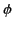is the potential due to a volume charge density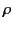within a volume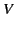and a surface charge density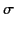on the conducting surface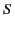bounding the volume, while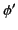is the potential due to another charge distribution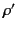and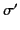(non-simultaneously occupying the same volume and the same surface, respectively), then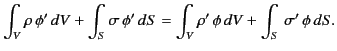3. Two infinite grounded parallel conducting planes are separated by a distance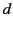. A point charge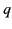is placed between the planes. Use Green's reciprocation theorem to prove that the total charge induced on one of the planes is equal to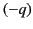times the fractional perpendicular distance of the point charge from the other plane. [Hint: Choose as your comparison electrostatic problem with the same surfaces one whose charge densities and potential are known and simple.]

4. Consider two insulated conductors, labeled 1 and 2. Let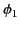be the potential of the first conductor when it is uncharged and the second conductor holds a charge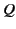. Likewise, let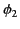be the potential of the second conductor when it is uncharged and the first conductor holds a charge. Use Green's reciprocation theorem to demonstrate that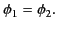5. Consider two insulated spherical conductors. Let the first have radius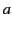. Let the second be sufficiently small that it can effectively be treated as a point charge, and let it also be located a distance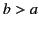from the center of the first. Suppose that the first conductor is uncharged, and that the second carries a charge. What is the potential of the first conductor? [Hint: Consider the result proved in Exercise 1.]

6. Consider a set of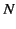conductors distributed in a vacuum. Suppose that the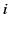th conductor carries the charge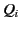and is at the scalar potential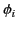. It follows from the linearity of Maxwell's equations and Ohm's law that a linear relationship exists between the potentials and the charges: that is,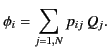Here, the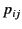are termed the coefficients of potential. Demonstrate that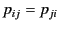for all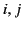. [Hint: Consider the result proved in Exercise 1.] Show that the total electrostatic potential energy of the charged conductors is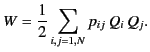7. Demonstrate that the Green's function for Poisson's equation in two dimensions (i.e.,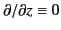) is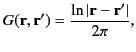where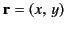, et cetera. Hence, deduce that the scalar potential field generated by the two-dimensional charge distribution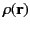is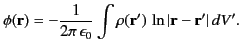8. A electric dipole of fixed moment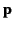is situated at position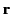in a non-uniform external electric field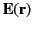. Demonstrate that the net force on the dipole can be written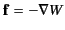, where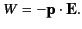9. Demonstrate that the electric field generated by an electric dipole of dipole momentis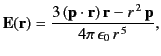whererepresents vector displacement relative to the dipole. Show that the potential energy of an electric dipole of moment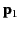in the electric field generated by a second dipole of moment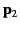is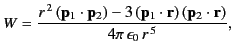whereis the displacement of one dipole from another.

10. Show that the torque on an electric dipole of momentin a uniform external electric field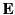is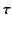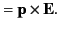Hence, deduce that the potential energy of the dipole is11. Consider two coplanar electric dipoles with their centers a fixed distance apart. Show that if the angles the dipoles make with the line joining their centers are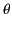and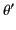, respectively, andis held fixed, then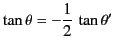in equilibrium.Next: Potential Theory Up: Electrostatic Fields Previous: Dirichlet Green's Function for
Richard Fitzpatrick 2014-06-27